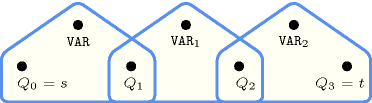## 5.154. equivalent

Origin

Logic

Constraint

$\mathrm{𝚎𝚚𝚞𝚒𝚟𝚊𝚕𝚎𝚗𝚝}\left(\mathrm{𝚅𝙰𝚁},\mathrm{𝚅𝙰𝚁𝙸𝙰𝙱𝙻𝙴𝚂}\right)$

Synonym

$\mathrm{𝚎𝚚}$.

Arguments
 $\mathrm{𝚅𝙰𝚁}$ $\mathrm{𝚍𝚟𝚊𝚛}$ $\mathrm{𝚅𝙰𝚁𝙸𝙰𝙱𝙻𝙴𝚂}$ $\mathrm{𝚌𝚘𝚕𝚕𝚎𝚌𝚝𝚒𝚘𝚗}\left(\mathrm{𝚟𝚊𝚛}-\mathrm{𝚍𝚟𝚊𝚛}\right)$
Restrictions
 $\mathrm{𝚅𝙰𝚁}\ge 0$ $\mathrm{𝚅𝙰𝚁}\le 1$ $|\mathrm{𝚅𝙰𝚁𝙸𝙰𝙱𝙻𝙴𝚂}|=2$ $\mathrm{𝚛𝚎𝚚𝚞𝚒𝚛𝚎𝚍}$$\left(\mathrm{𝚅𝙰𝚁𝙸𝙰𝙱𝙻𝙴𝚂},\mathrm{𝚟𝚊𝚛}\right)$ $\mathrm{𝚅𝙰𝚁𝙸𝙰𝙱𝙻𝙴𝚂}.\mathrm{𝚟𝚊𝚛}\ge 0$ $\mathrm{𝚅𝙰𝚁𝙸𝙰𝙱𝙻𝙴𝚂}.\mathrm{𝚟𝚊𝚛}\le 1$
Purpose

Let $\mathrm{𝚅𝙰𝚁𝙸𝙰𝙱𝙻𝙴𝚂}$ be a collection of 0-1 variables ${\mathrm{𝚅𝙰𝚁}}_{1},{\mathrm{𝚅𝙰𝚁}}_{2}$. Enforce $\mathrm{𝚅𝙰𝚁}=\left({\mathrm{𝚅𝙰𝚁}}_{1}⇔{\mathrm{𝚅𝙰𝚁}}_{2}\right)$.

Example
 $\left(1,〈0,0〉\right)$ $\left(0,〈0,1〉\right)$ $\left(0,〈1,0〉\right)$ $\left(1,〈1,1〉\right)$
Symmetries
• Items of $\mathrm{𝚅𝙰𝚁𝙸𝙰𝙱𝙻𝙴𝚂}$ are permutable.

• All occurrences of 0 in $\mathrm{𝚅𝙰𝚁}$ and in $\mathrm{𝚅𝙰𝚁𝙸𝙰𝙱𝙻𝙴𝚂}.\mathrm{𝚟𝚊𝚛}$ can be set to 1.

Arg. properties

Functional dependency: $\mathrm{𝚅𝙰𝚁}$ determined by $\mathrm{𝚅𝙰𝚁𝙸𝙰𝙱𝙻𝙴𝚂}$.

Counting
 Length ($n$) 2 3 4 5 6 7 8 Solutions 4 0 0 0 0 0 0

Number of solutions for $\mathrm{𝚎𝚚𝚞𝚒𝚟𝚊𝚕𝚎𝚗𝚝}$: domains $0..n$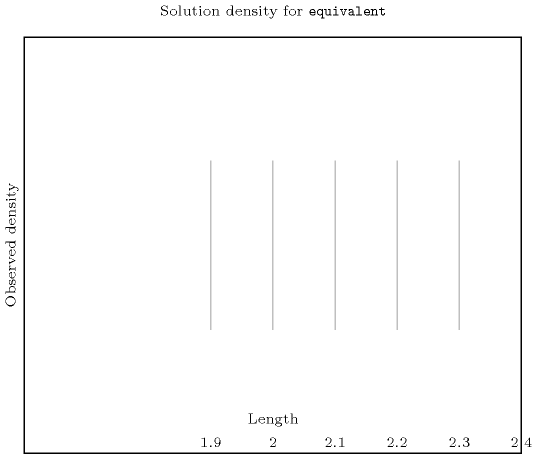Length ($n$)2
Total4
 Parameter value

02
12

Solution count for $\mathrm{𝚎𝚚𝚞𝚒𝚟𝚊𝚕𝚎𝚗𝚝}$: domains $0..n$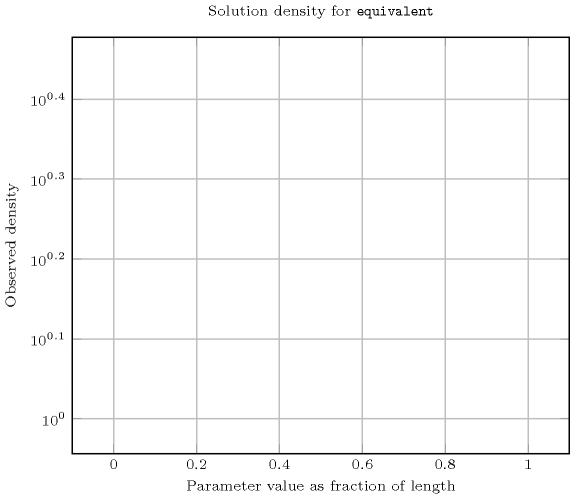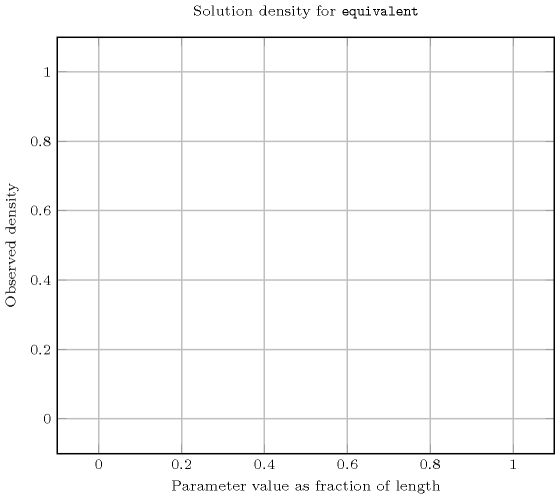Systems

ifOnlyIf in Choco, rel in Gecode, eqbool in JaCoP, #<=> in SICStus.

Figure 5.154.1 depicts the automaton associated with the $\mathrm{𝚎𝚚𝚞𝚒𝚟𝚊𝚕𝚎𝚗𝚝}$ constraint. To the first argument $\mathrm{𝚅𝙰𝚁}$ of the $\mathrm{𝚎𝚚𝚞𝚒𝚟𝚊𝚕𝚎𝚗𝚝}$ constraint corresponds the first signature variable. To each variable ${\mathrm{𝚅𝙰𝚁}}_{i}$ of the second argument $\mathrm{𝚅𝙰𝚁𝙸𝙰𝙱𝙻𝙴𝚂}$ of the $\mathrm{𝚎𝚚𝚞𝚒𝚟𝚊𝚕𝚎𝚗𝚝}$ constraint corresponds the next signature variable. There is no signature constraint.
##### Figure 5.154.1. Automaton of the $\mathrm{𝚎𝚚𝚞𝚒𝚟𝚊𝚕𝚎𝚗𝚝}$ constraint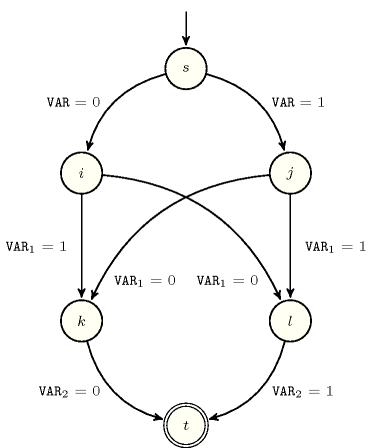##### Figure 5.154.2. Hypergraph of the reformulation corresponding to the automaton of the $\mathrm{𝚎𝚚𝚞𝚒𝚟𝚊𝚕𝚎𝚗𝚝}$ constraint